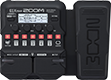# slipknot

Discussion in 'Zoom G1/G1X Four' started by Rozeane, Dec 24, 2022.

1. slipknotDevice: Zoom G1X Four
Firmware: 2.00

Name on device: SLIPKCNOT
Optimized for: Phones/Speaker

Effects chain:This straight rock sound is inspired by the sound of Jimmy Page with Led Zeppelin.

Effect: "TS Drive" (Overdrive / Distortion), active - "yes"
"Gain" = 0
"Boost" = Off
"Tone" = 50
"Volume" = 92

Effect: "ORG120" (Amp simulator), active - "yes"
"Input" = HI
"Color" = 1
"Bass" = 10
"Treble" = 60
"Presence" = 50
"Gain" = 70
"Volume" = 80

Effect: "MS4x12" (Cabinet), active - "yes"
"MIC" = ON
"D57: D421" = 55
"Hi" = 75
"Lo" = 10

Effect: "ParaEQ" (Filter), active - "no"
"Frequency" = 125Hz
"Q" = 0.6
"Gain" = 0.0
"Volume" = 80

Effect: "Gt GEQ 7" (Filter), active - "yes"
"100" = 0.0
"200" = 0.0
"400" = 0.5
"800" = 0.5
"1.6k" = 1.5
"3.2k" = 1.0
"6.4k" = 0.0
"VOL" = 80

Patch Volume: 58